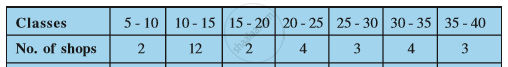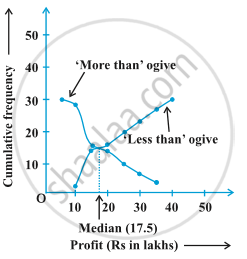Karnataka Secondary Education Examination BoardKarnataka SSLC

# Graphical Representation of Cumulative Frequency Distribution

## Notes

In this concept we will study about Relation of Ogive and Median. These graphical representation of the frequency distribution are called Ogives. Actual limits are on the x-axis and cumulative frequencies on the y-axis.

Ogive is also known as cumulative frequency distribution.

You will be asked to draw either a less than frequency ogive or more than frequency ogive.

Example: The annual profits earned by 30 shops of a shopping complex in a locality give rise to the following distribution :Convert the distribution above to a less than type cumulative frequency distribution and also to a more than type cumulative frequency distribution, and draw its ogive.

1) Less than type cumulative frequency table

 Classes cf Less than 10 2 Less than 15 14 Less than 20 16 Less than 25 20 Less than 30 23 Less than 35 27 Less than 40 30

2) More than type cumulative frequency table

 Classes cf More than 5 30 More than 10 28 More than 15 16 More than 20 14 More than 25 10 More than 30 7 More than 35 3

The graphical representation of both the ogives will beHere, N/2= 30/2= 15

Now, we will take 15 on y axis and draw a perpendicular line that touches the curve, and from the point where the perpendicular touches the curve draw a perpendicular that touches the x axis, the point at which the perpendicular touches the x axis is the median.

Thus, here the Median is 17.5

If you would like to contribute notes or other learning material, please submit them using the button below.